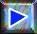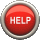﻿ The mole: tutorial 1# The mole

## Tutorial 1How many are A) $2$ moles of helium atoms ? B) $1.2 \cdot 10^{-13}$ moles of water molecules ?

For answers, use (possibly several times) the arrows ↑ Down! and ↓ Up! Complete please this question before moving on to the next one!

2 moles of helium atoms are $2 \cdot 6.023 \cdot 10^{23} = 1.205 \cdot 10^{24}$ helium atoms.

$1.2 \cdot 10^{-13}$ moles of water molecules are $1.2 \cdot 10^{-13} \cdot 6.023 \cdot 10^{23} = 7.408 \cdot 10^{10}$ water molecules.